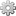# LaTeX for Numbered FiguresProvides the User Interface Extensions Points to integrate Numbered Figures with the Latex Extension
Recommended
 Compatibility 14.10+

Installable with the Extension Manager

# Description

This extension is only needed if you wish to use Numbered Figures Application in combination with LaTeX.

It allows to take into account the counters configuration during LaTeX export.

# Example

• A block type graph is configured
• Two inline types theorem and lemma are configured
• A counter math containing theorem and lemma is configured
• Numbered Figures are activated on the page
{{figure type="graph"}}
[[image:Polynomial_of_degree_three.png]]

{{figureCaption}}
Polynomial of degree three
{{/figureCaption}}
{{/figure}}

{{figure type="theorem"}}
{{figureCaption}}
Pythagorean theorem
{{/figureCaption}}

Let ##f## be a function whose derivative exists in every point, then ##f## is  a continuous function.
{{/figure}}

{{figure type="lemma"}}
Given two line segments whose lengths are ##a## and ##b## respectively there is a real number ##r## such that ##b=ra##.
{{/figure}}

## XWiki Page result## LaTeX Export result# Export Technical Details

Everything documented in LaTeX for the Figure Macro holds, but two things are adapted to take into account counters configuration.

## Block Figure Export

When figures of style block share a counter, the prelude is adapted. The first type of the counter will be declared as a floating environment, and the following types are skipped.
Then, during the FigureBlock generation, all the types of the same counter will use the identifier of the first type as their environment so that they share the same counter.
But, all the FigureCaptionBlock elements contained in a given FigureBlock are overridden with the caption label corresponding to their actual type.
Therefore, the counter is shared between the figure types, but their label matched their actual type.
For instance, assuming graph and plot shares the same counter, the following latex will be produced for a document containing two figures respectivelly of :

% Prelude:
\DeclareFloatingEnvironment{graph}
% No floating environment for 'plot'.
\begin{document}

\begin{graph}[h]
% Graph content...

\captionsetup{name=Graph}

\caption[Graph caption]{Graph caption} % Graph 1: Graph caption
\end{graph}

\begin{graph}[h] % Figure of type plot using the graph environment as it is the first figure type of its counter
% Plot content...

\captionsetup{name=Plot} % Overridden caption label

\caption[Plot caption]{Plot caption} % Plot 2: Graph caption (the counter is incremented here)
\end{graph}
\end{document}

## Inline Figure Export

When figures of style inline share a counter, the prelude is adapted. The first figure type of the counter will be declared as usual, and all the following figure types of the same counter will have an additional parameters reference the first figure type.
In the case of the example above, the prelude is:

\newtheorem{lemma}{Lemma}
\newtheorem{theorem}[lemma]{Theorem} % The second type 'theorem' references the first type 'lemma' so that they share a counter

The rest of the generation stays unchanged.

# Prerequisites & Installation Instructions

We recommend using the Extension Manager to install this extension (Make sure that the text "Installable with the Extension Manager" is displayed at the top right location on this page to know if this extension can be installed with the Extension Manager).

You can also use the manual method which involves dropping the JAR file and all its dependencies into the WEB-INF/lib folder and restarting XWiki.

# Dependencies

Dependencies for this extension (org.xwiki.contrib.numbered.content:application-numbered-content-figures-latex 1.9.2):

Tags: